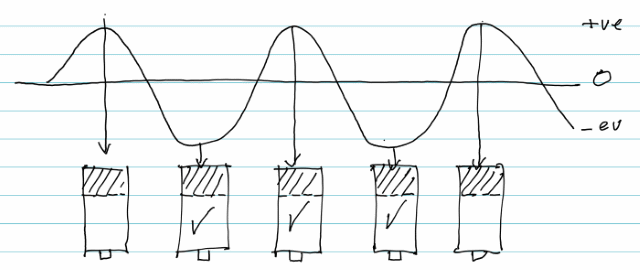# Detecting a Waggle

I spent a long while thinking and discussing with others how a waggle could be detected. Two I guess opposite ideas were proposed:

1. Sine wave mapping : This involves smoothing in the incoming data of “delta X” values and then mapping a sine wave to it, if the two overlap within a degree of tolerance, then a waggle exists.
2. Simple Threshold : This is simply, if the “delta X” value passes a set threshold then we are in a waggle.

There are issues with both approaches, firstly the Sine wave mapping sounds good, but if the user speeds up the waggle then the frequency of the wave changes, and the mapping doesn’t work. Secondly the simple threshold value doesn’t work, we’d need multiples of the them, as the “delta X” values go from positive to negative. We’d also need to account of the time difference between the two. So it is not as simple as we first thought. Another solution is required.

# Temporarily a bit True

When a bit is roughly true, some of the time.Imagine a jar, the top of the jar is completely open and sand can flow into it. The bottom of the jar has a small exit through which the sand must pass. Now imagine that upon this jar there is a line, a threshold. If the sand is above this line, then the jar is said to be true. If the sand is below this line, then the Jar is said to be false.

If we used, say 5 sand jars to record the waggle thresholds we would record in the first jar the fact that the “delta X” threshold has been passed by filling the jar up to it’s brim. We’d then move on to the next Jar and wait for the “delta X” to pass the opposite threshold. If the first jar was filled because the “delta X” value was above the positive threshold, then the next jar will only be filled if the “delta X” threshold passes the negative threshold.We would continue to do this for each of the 5 jars, when we reach the last jar, we would move back to the first and repeat.

If we were to watch all the jars and if any three of the five jars where above the threshold, and true. Then we could say that ‘Yes, we are in a waggle’.

This approach is the time dependant threshold approach. Right now it’s a concept, and now I have to code it and check to see if works…

This entry was posted in mind-flip, work and tagged , , , , , on by .